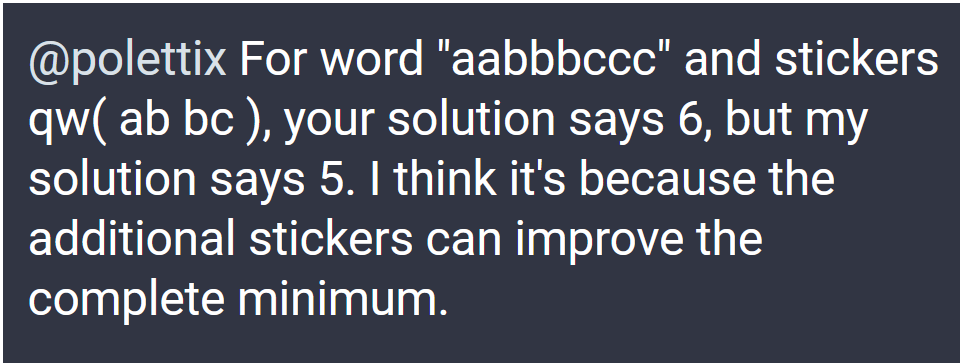TL;DR

E. Choroba tested my solution and found bugs.

In particular:I ended up finding two bugs.

The first was about missing to calculate the right amount of minimum stickers needed. In the example:

• letter `a` appears twice, which means we need two `ab` stickers;
• letter `c` appears thrice, which means we need three `bc` stickers.

The solution is calculating the right amount by dividing the amount that’s needed by the amount that’s provided by each sticker. In Perl terms:

``````# check for a viable solution and set the bare minimum
my %minimum;
for my \$letter (keys(%needed)) {
my \$alternatives = \$provided{\$letter}
or return 0; # no viable source
if (scalar(keys(\$alternatives->%*)) == 1) { # one viable source only
my (\$word, \$units) = \$alternatives->%*;
my \$amount = int(\$needed{\$letter} / \$units)
+ (\$needed{\$letter} % \$units ? 1 : 0);
my \$amount = \$units;
\$minimum{\$word} = \$amount
if (! exists(\$minimum{\$word})) || (\$minimum{\$word} < \$amount);
}
}
``````

On the other hand, I already knew that there was at least another error. In theory, calculating the minimum etc. was not strictly needed, as the final step with the breadth-first search should have sufficed. Yet it didn’t, so it had to have a bug too.

It turned out that I was wasting useful pieces of stickers while doing the search, i.e. I was only using specific parts without using the rest to cover for other letters needs. This required turning the algotithm a bit to first find out all words that can possibly advance a little towards a solution, then iterate over those words by taking complete advantage of all provided letters. In Raku terms:

``````sub complete-minimum (%minimum is copy, %needed is copy, %provided) {
my @queue = {needed => %needed, minimum => %minimum},;
while @queue {
my \$frame = @queue.shift;
my \$needed = \$frame<needed>;
my \$minimum = \$frame<minimum>;

my %words = \$needed.keys.map({ %provided{\$_}.keys }).flat.map({ \$_ => 1 });
for %words.keys -> \$source {
my %nmin  = %\$minimum;
%nmin{\$source}++;
my %nneed = %\$needed;
for %nneed.keys -> \$letter {
%nneed{\$letter} -= %provided{\$letter}{\$source} // 0;
%nneed{\$letter}:delete if %nneed{\$letter} <= 0;
}
return %nmin if %nneed.keys.elems == 0;
@queue.push: {needed => %nneed, minimum => %nmin};
}
}
return ();
}
``````

Thanks E. Choroba!

Comments? Octodon, , GitHub, Reddit, or drop me a line!